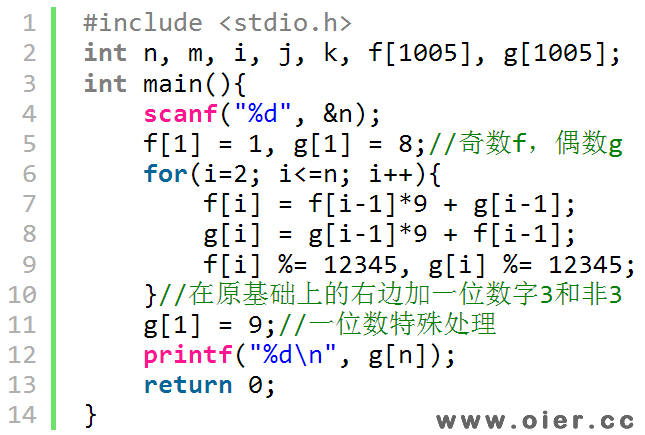SSOJ2353位数问题
895+

## 样例输入

2

## 样例输出

73

1<=n<=1000

# 程序实现f[n] + g[n] = 10 * (f[n-1] + g[n-1]) = 10 * 10 * (f[n-2] + g[n-2]) = … = 10 ^ (n-1) * (f + f) = 10 ^ (n-1) * 9

f[n] – g[n] = 8 * (f[n-1] – g[n-1]) = 8 * 8 * (f[n-2] – g[n-2]) = … = 8 ^ (n-1) * (f – f) = 8 ^ (n-1) * (-7)